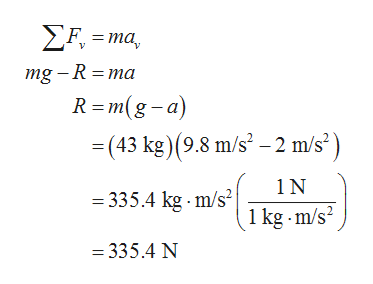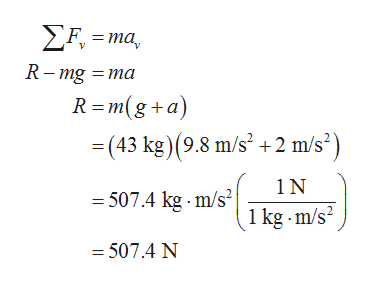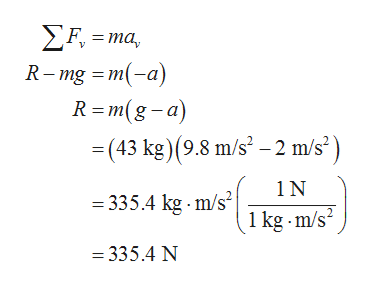Question
18 views

A child of mass 43 kg stands on a scale inside an elevator.

Select one or more:

A. The scale would show his weight as 335.40 N when the elevator accelerates downward at 2 m/s2.
B. The scale would show his weight as 507.4 N when the elevator accelerates upward at 2 m/s2.
C. The scale would show his weight as 335.40 N when the elevator accelerates upward at 2 m/s2.
D. The scale would show his weight as 335.40 N when the elevator decelerates upward at 2 m/s2.
E. The scale would show his weight as 421.4 N when the elevator is stationary.
F. The scale would show his weight as 335.4 N when the elevator decelerates upward at 2 m/s2.
G. The scale would show his weight as 421.40 N when the elevator moves with a constant velocity of 2.5 m/s.
check_circle

Step 1

Consider the acceleration of the elevator be a, and the speed of the elevator be v.

Apply Newton’s second law of the motion along the vertical direction, and solve for the normal reaction on the child, that is the weight shown by the scale, when the elevator accelerates downward at 2 m/s2.help_outlineImage TranscriptioncloseF=ma mg -R ma R =m(g-a) (43 kg) (9.8 m/s2-2 m/s2) 1N =335.4 kg.m/s2 1 kg m/s2 = 335.4 N fullscreen
Step 2

Apply Newton’s second law of the motion along the vertical direction, and solve for the normal reaction on the child, that is the weight shown by the scale, when the elevator accelerates upward at 2 m/s2.help_outlineImage TranscriptioncloseF=ma R-mg m R m(g+a) (43 kg) (9.8 m/s2 +2 m/s2) 1N =507.4 kg m/s 1 kg m/s2 507.4 N = fullscreen
Step 3

Apply Newton’s second law of the motion along the vertical direction, and solve for the normal reaction on t...help_outlineImage TranscriptioncloseF,ma R-mg a) R=m(g-a) =(43 kg) (9.8 m/s2-2 m/s2) 1N = 335.4 kg m/s 1 kg m/s2 =335.4 N fullscreen

### Want to see the full answer?

See Solution

#### Want to see this answer and more?

Solutions are written by subject experts who are available 24/7. Questions are typically answered within 1 hour.*

See Solution
*Response times may vary by subject and question.
Tagged in

### Physics